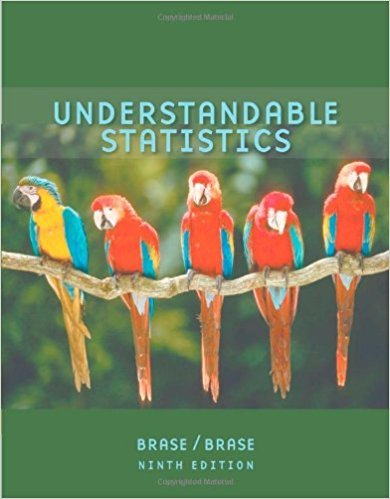×
×

# Solutions for Chapter 5.3: The Binomial Probability Distribution and Related Topics## Full solutions for Understandable Statistics | 9th Edition

ISBN: 9780618949922Solutions for Chapter 5.3: The Binomial Probability Distribution and Related Topics

Solutions for Chapter 5.3
4 5 0 315 Reviews
27
4
##### ISBN: 9780618949922

Chapter 5.3: The Binomial Probability Distribution and Related Topics includes 24 full step-by-step solutions. This expansive textbook survival guide covers the following chapters and their solutions. Since 24 problems in chapter 5.3: The Binomial Probability Distribution and Related Topics have been answered, more than 35618 students have viewed full step-by-step solutions from this chapter. This textbook survival guide was created for the textbook: Understandable Statistics, edition: 9. Understandable Statistics was written by and is associated to the ISBN: 9780618949922.

Key Statistics Terms and definitions covered in this textbook
• Alias

In a fractional factorial experiment when certain factor effects cannot be estimated uniquely, they are said to be aliased.

• Arithmetic mean

The arithmetic mean of a set of numbers x1 , x2 ,…, xn is their sum divided by the number of observations, or ( / )1 1 n xi t n ? = . The arithmetic mean is usually denoted by x , and is often called the average

• Average

See Arithmetic mean.

• Bayes’ theorem

An equation for a conditional probability such as PA B ( | ) in terms of the reverse conditional probability PB A ( | ).

• Block

In experimental design, a group of experimental units or material that is relatively homogeneous. The purpose of dividing experimental units into blocks is to produce an experimental design wherein variability within blocks is smaller than variability between blocks. This allows the factors of interest to be compared in an environment that has less variability than in an unblocked experiment.

• C chart

An attribute control chart that plots the total number of defects per unit in a subgroup. Similar to a defects-per-unit or U chart.

• Central tendency

The tendency of data to cluster around some value. Central tendency is usually expressed by a measure of location such as the mean, median, or mode.

• Conditional mean

The mean of the conditional probability distribution of a random variable.

• Conditional probability distribution

The distribution of a random variable given that the random experiment produces an outcome in an event. The given event might specify values for one or more other random variables

• Consistent estimator

An estimator that converges in probability to the true value of the estimated parameter as the sample size increases.

• Counting techniques

Formulas used to determine the number of elements in sample spaces and events.

• Covariance matrix

A square matrix that contains the variances and covariances among a set of random variables, say, X1 , X X 2 k , , … . The main diagonal elements of the matrix are the variances of the random variables and the off-diagonal elements are the covariances between Xi and Xj . Also called the variance-covariance matrix. When the random variables are standardized to have unit variances, the covariance matrix becomes the correlation matrix.

• Cumulative normal distribution function

The cumulative distribution of the standard normal distribution, often denoted as ?( ) x and tabulated in Appendix Table II.

• Decision interval

A parameter in a tabular CUSUM algorithm that is determined from a trade-off between false alarms and the detection of assignable causes.

• Defect concentration diagram

A quality tool that graphically shows the location of defects on a part or in a process.

• Design matrix

A matrix that provides the tests that are to be conducted in an experiment.

• Discrete distribution

A probability distribution for a discrete random variable

• F-test

Any test of signiicance involving the F distribution. The most common F-tests are (1) testing hypotheses about the variances or standard deviations of two independent normal distributions, (2) testing hypotheses about treatment means or variance components in the analysis of variance, and (3) testing signiicance of regression or tests on subsets of parameters in a regression model.

• Generating function

A function that is used to determine properties of the probability distribution of a random variable. See Moment-generating function

• Generator

Effects in a fractional factorial experiment that are used to construct the experimental tests used in the experiment. The generators also deine the aliases.

×